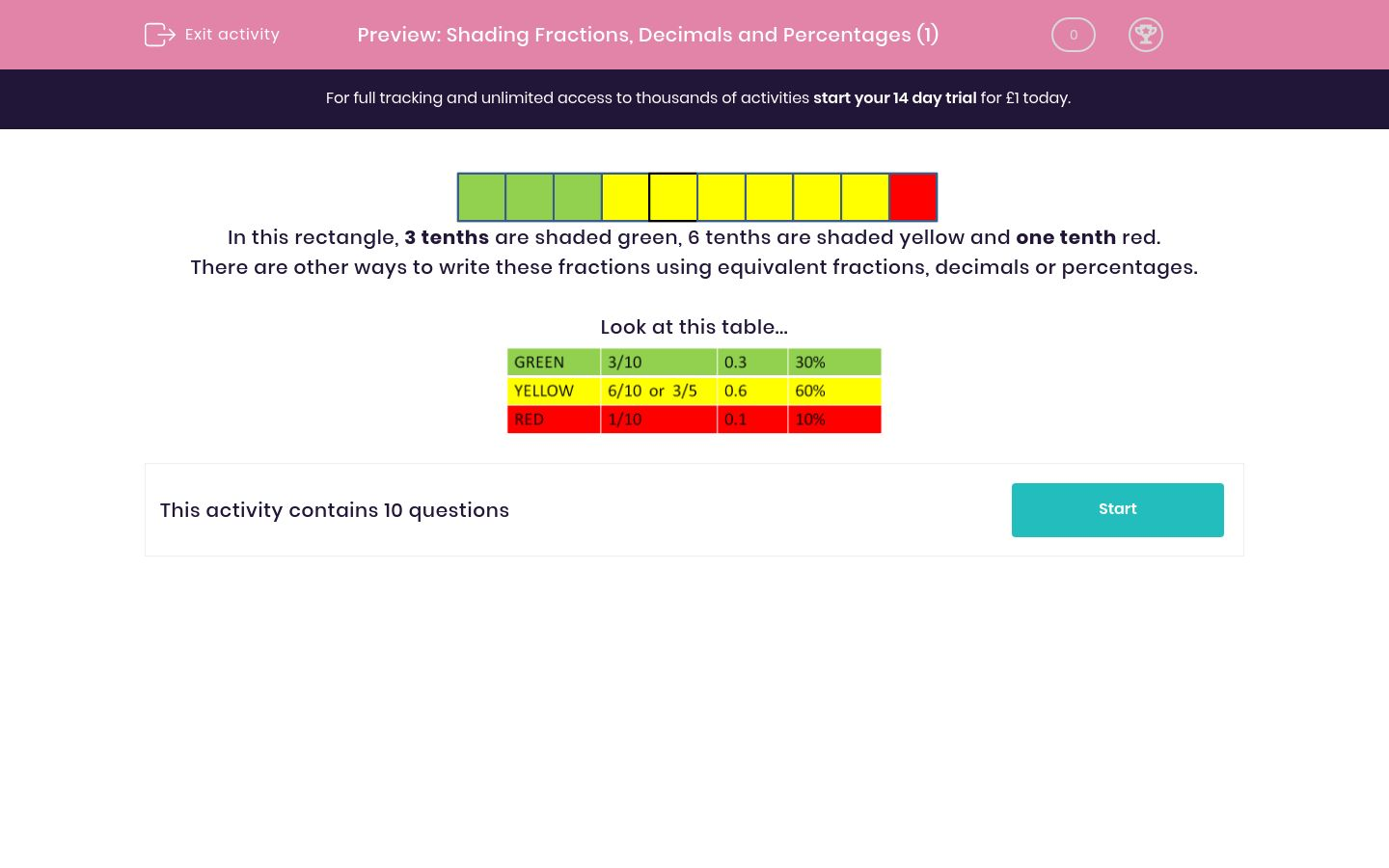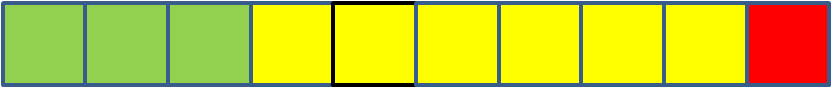# Shading Fractions, Decimals and Percentages (1)

In this worksheet, students identify the fraction, decimal or percentage shaded in a rectangle.Key stage:  KS 2

Curriculum topic:   Maths and Numerical Reasoning

Curriculum subtopic:   Decimals

Difficulty level:### QUESTION 1 of 10In this rectangle, 3 tenths are shaded green, 6 tenths are shaded yellow and one tenth red.

There are other ways to write these fractions using equivalent fractions, decimals or percentages.

Look at this table...---- OR ----